Convert Minutes To Seconds  by  Alice Akinyi

6

Time is made of years, months,weeks ,days hours minutes and seconds.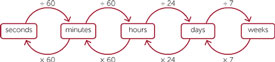• 60seconds= 1 minute

• 60minutes= 1 hour

• 3600seconds= 1hour

• 24hours= 1day

• 7days= 1weeks

• 4 weeks=1month

• 12 months= 1 year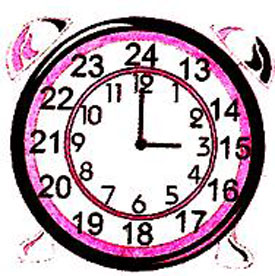24hours=1 day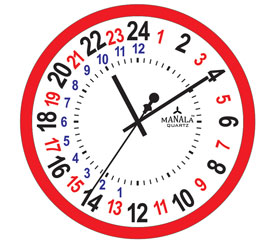#### How many seconds are in the following minutes?

• 1minute

• 5minutes

• 10minutes

• 60 minutes

Solution

(a) 1minute
1minute=60 seconds
Therefore 1minute =  1 ×   60
= 60seconds

( b) 5minutes
1minute= 60 seconds
5minutes= 5x60
300 seconds

(C) 10minutes
1minutes =60 seconds
10 minutes = 10 x 60
= 600 seconds

( d ) 60 minutes
1minute= 60 seconds
60minutes= 60x 60
=3600 seconds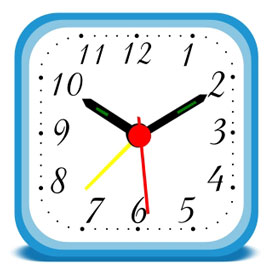#### CONVERT SECONDS TO MINUTES

1 minute= 60 seconds

Example 1
Convert   120seconds into minutes
120 = 120  =  2 minutes
60

Example 2
Convert 420 seconds to minutes
1minute= 60 seconds
420= 420    =7minutes
60

Example 3
Convert 3600 into minutes

60 seconds =1 minute
3600=  3600  =  6o minutes
60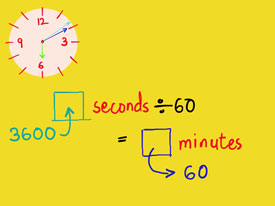• 240

• 300

• 720

• 90

• 360